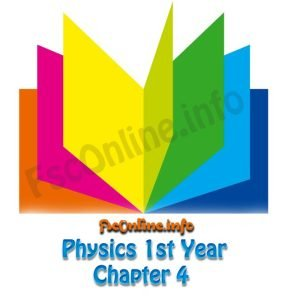Latest

# Chapter 4 Notes | Physics 1st Year

## “Work And Energy”

1. Define the term work. Also derive the expression of work done by a variable force.2. Show that the work done in earth’s gravitational field is independent of path followed.
3. Show that work done in a close path is zero or Show that earth’s gravitational field is conservative field.
4. Define the term power. Show that power is the dot product of force and velocity.
5. Show that 1kWh = 3.6 MJ.
6. Define the term energy. Describe the different types of mechanical energy.
7. State the work energy principle. Also derive it mathematical form.
8. What do you know about absolute potential energy? Also derive its expression.
9. Define the term escape velocity. Also derive its expression.
10. Describe the inter-conversation of potential energy into kinetic energy for a free falling object.
11. State the law of conservation of energy.

Categories: Fsc Part 1,Notes,Physics